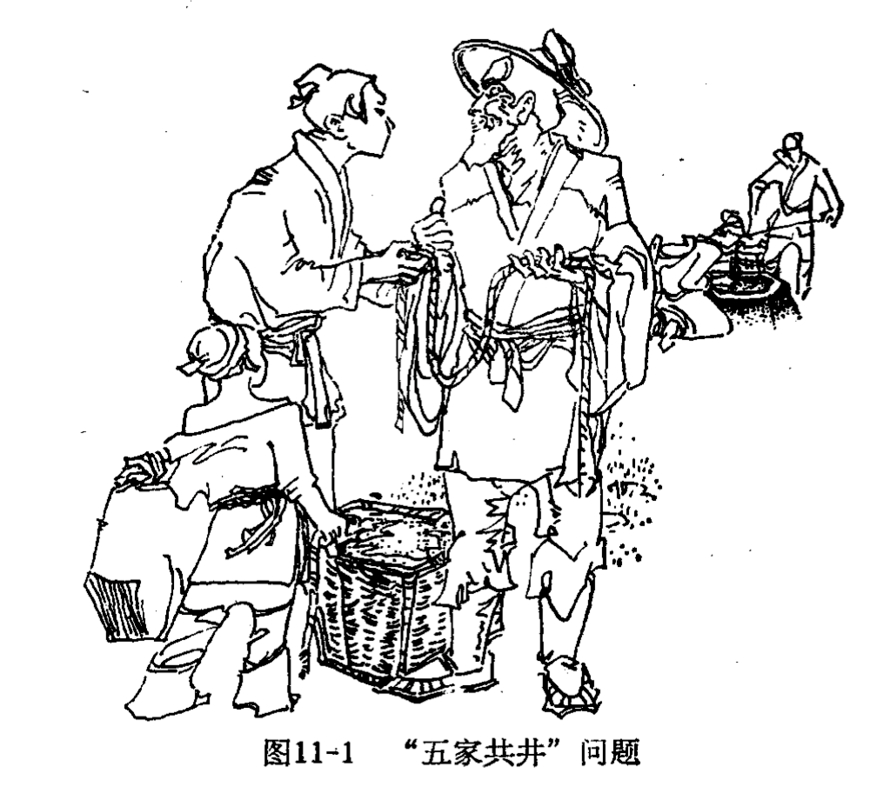【答案】 其实这是一个不定方程组问题，设井深为 $l$ 个单位长度，甲、乙、丙、丁、戊绳长分别为 $x, y, z, w, u$ 个长度单位，那么依题意得方程组
$$\left\{\begin{array}{l} l=2 x+y, \\ l=3 y+z, \\ l=4 z+w, \\ l=5 w+u, \\ l=6 u+x, \end{array}\right.$$

(6)代入( 2 )得
$$l=3(l-2 x)+z, \Longrightarrow z=6 x-2 l$$
(7) 代入( 3 )得
$$l=4(6 x-2 l)+w, \Longrightarrow w=9 l-24 x$$
(8)代入( 4 )得
$$l=5(9 l-24 x)+u, \Longrightarrow u=120 x-44 l$$
(9) 代入(5)得
$$l=6(120 x-44 l)+x, \Longrightarrow 265 l=721 x \text { (10) }$$

$$y=191, z=148, w=129, u=76 .$$